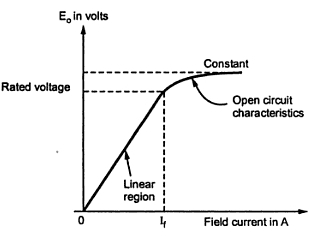### Characteristics of D.C. Generators

The d.c. generators have following characteristics in general,
1) Magnetization characteristics
1.1 Magnetization Characteristics
This characteristics is the graph of generated no load voltage E against the field current If, when speed of, generator is maintained constant. As it is plotted without load with open output terminals it is also called No load characteristics or Open circuit characteristics.
Eo Vs Iis magnetization characteristics
Where  Eo = No load induced e.m.f.
But for generator,
E = (ΦPNZ)/(60A)
...     E α   Φ                 with (PNZ) / (60A)  constant
...     α   If                    as   Φ  α   If
Thus induced e.m.f. increases directly as I increases. But after certain I core gets saturated and flux also remain constant though Iincreases. Hence after saturation, voltage also remains constatnt.
Note : Thus characteristics is linear till saturation and after that bends such that voltage remains constant though I increases.
The characteristics is shown in the Fig. 1.Fig. 1  Magnetization characteristics

Now the induced e.m.f. also varies with speed.
Actually,             E   α   N Φ
So if magnetization characteristics for various speeds are plotted we will get family of parallel characteristics as shown in the Fig. 2. For lower speeds, generated voltages are less so characteristics for lower speeds are below the characteristics for higher speeds.Fig. 2